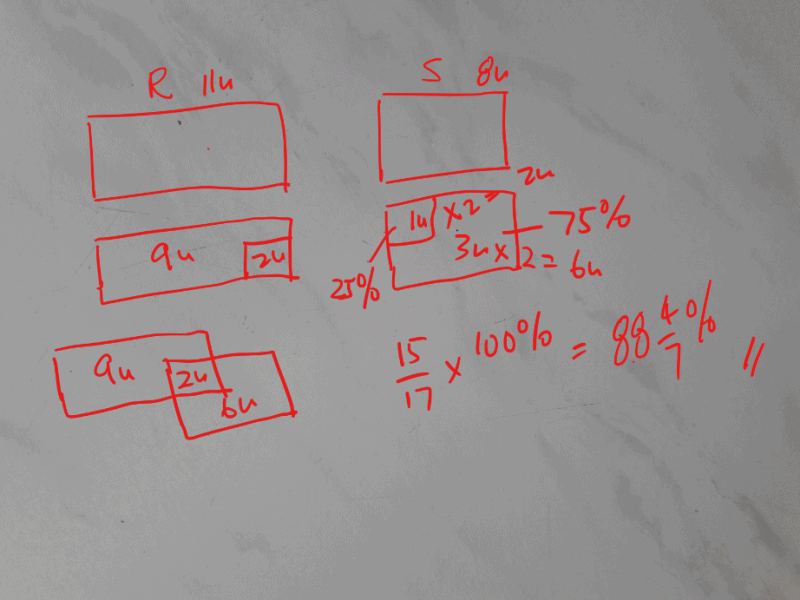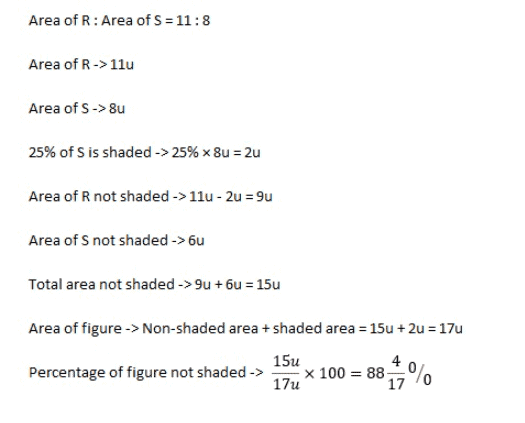# Question

The figure below, not drawn to scale is made up of two overlapping shaped, rectangle R and square S. The ratio of the total area of r to the total area of s is 11:8. 25% of the total area of S is shaded. What percantage of the whole figure is UNSHADED? give your answer as a mixed number in its simplest form